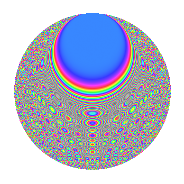# Properties

 Label 1003.2.gLevel 1003 Weight 2 Character orbit g Rep. character $$\chi_{1003}(60,\cdot)$$ Character field $$\Q(\zeta_{8})$$ Dimension 344 Sturm bound 180

# Related objects

## Defining parameters

 Level: $$N$$ = $$1003 = 17 \cdot 59$$ Weight: $$k$$ = $$2$$ Character orbit: $$[\chi]$$ = 1003.g (of order $$8$$ and degree $$4$$) Character conductor: $$\operatorname{cond}(\chi)$$ = $$17$$ Character field: $$\Q(\zeta_{8})$$ Sturm bound: $$180$$

## Dimensions

The following table gives the dimensions of various subspaces of $$M_{2}(1003, [\chi])$$.

Total New Old
Modular forms 368 344 24
Cusp forms 352 344 8
Eisenstein series 16 0 16

## Trace form

 $$344q - 8q^{5} - 16q^{6} + O(q^{10})$$ $$344q - 8q^{5} - 16q^{6} - 16q^{10} + 8q^{11} - 4q^{15} - 320q^{16} + 32q^{18} - 32q^{19} + 16q^{20} - 48q^{22} + 16q^{24} - 16q^{26} - 48q^{27} + 16q^{28} + 8q^{29} - 16q^{31} + 40q^{32} - 48q^{33} + 64q^{34} - 64q^{35} + 64q^{36} - 32q^{37} + 16q^{39} + 32q^{40} - 8q^{41} + 96q^{42} + 16q^{43} + 64q^{44} - 52q^{45} - 80q^{46} - 40q^{49} - 80q^{50} + 16q^{51} + 64q^{52} + 8q^{53} - 64q^{54} - 56q^{56} - 56q^{61} + 16q^{62} + 16q^{63} + 72q^{65} - 16q^{66} - 64q^{67} + 40q^{68} + 112q^{69} + 56q^{70} - 32q^{71} + 80q^{73} - 96q^{74} - 8q^{75} + 120q^{76} - 80q^{77} - 48q^{78} - 8q^{80} + 64q^{82} + 32q^{83} - 96q^{84} - 64q^{85} + 32q^{86} + 8q^{88} - 32q^{90} + 80q^{91} + 192q^{92} - 152q^{93} - 16q^{94} + 8q^{95} + 16q^{96} - 72q^{99} + O(q^{100})$$

## Decomposition of $$S_{2}^{\mathrm{new}}(1003, [\chi])$$ into newform subspaces

The newforms in this space have not yet been added to the LMFDB.

## Decomposition of $$S_{2}^{\mathrm{old}}(1003, [\chi])$$ into lower level spaces

$$S_{2}^{\mathrm{old}}(1003, [\chi]) \cong$$ $$S_{2}^{\mathrm{new}}(17, [\chi])$$$$^{\oplus 2}$$